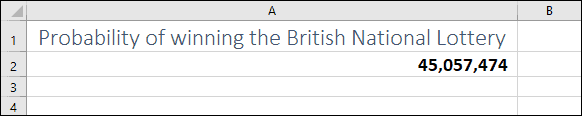# How to use factorial (!) in Excel

## What is a factorial?

A factorial calculates the ‘product’ of all numbers less than or equal to a value. For example, the factorial of 5 would be:

5x4x3x2x1=120

In mathematical notation, factorials are usually indicated with an exclamation mark. 5! would indicate the factorial of 5.

Factorials are typically used to calculate a number of possible combinations (or permutations). For example, the British National Lottery sells lottery tickets that contains 6 numbers from 1 to 59. The mathematical formula to calculate the total possible combinations of numbers is:

59!/(6!*(59-6)!)

This results in a value of 45,057,474, showing that a single ticket has a 1 in 45,057,474 chance of winning the British National Lottery!

## Factorials in Excel

Because the exclamation mark is the symbol for a factorial you might expect it to be recognized by Excel, but you will get an error message if you try to enter a formula such as =5!.

To calculate factorials in excel you must use the FACT function.

=FACT(5) would calculate the factorial of 5 in Excel.

If you’re unfamiliar with Excel formulas and functions you could benefit greatly from our completely free Basic Skills E-book.

Many more advanced functions are explained in depth in our Expert Skills Books and E-books.

## Calculating the probability of winning the lottery in Excel

Now that you know how to use the FACT function, it’s a simple matter to translate the mathematical formula shown above into an Excel formula:

=FACT(59)/(FACT(6)*FACT(59-6))